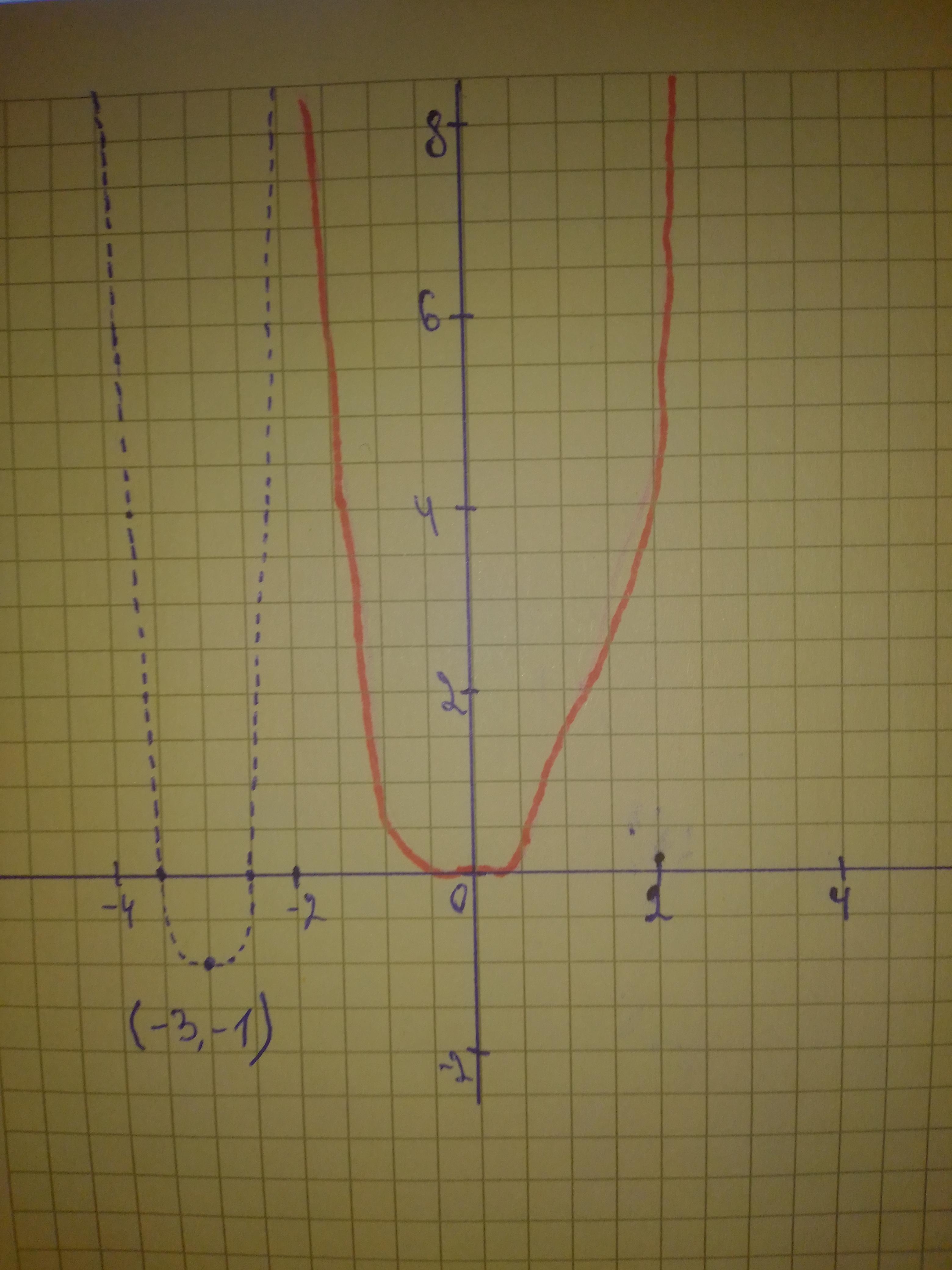# a) Identify the parameters a, k, d, and c in the polynomial function y=frac{1}{3}[-2(x+3)]^{4}-1. Describe how each parameter transforms the base funcSinead Mcgee 2021-02-26 Answered
a) Identify the parameters a, k, d, and c in the polynomial function $y=\frac{1}{3}{\left[-2\left(x+3\right)\right]}^{4}-1$. Describe how each parameter transforms the base function $y={x}^{4}$. b) State the domain and range, the vertex, and the equation of the axis of symmetry of the transformed function. c) Describe two possible orders in which the transformations can be applied to the graph of $y={x}^{4}$ to produce the graph of $y=\frac{1}{3}{\left[-2\left(x+3\right)\right]}^{4}-1$. d) Sketch graphs of the base function and the transformed function on the same set of axes.
You can still ask an expert for help

• Questions are typically answered in as fast as 30 minutes

Solve your problem for the price of one coffee

• Math expert for every subject
• Pay only if we can solve itFaiza Fuller

Step1
a) The parameters:
$a=\frac{1}{3},k=2,d=3,c=-1$
The function has a vertical compression by factor $\frac{1}{3}$, reflect in the y-axis, a horizontal compression by factor 2, horizontal left translated by 3 units, and vertical translated by 1 unit down.
b) Domain: $\left(-\mathrm{\infty },\mathrm{\infty }\right)$, Range: $\left[-1,\mathrm{\infty }\right)$
$\left(-3,-1\right)$, Line of symmetry: $x=-3$